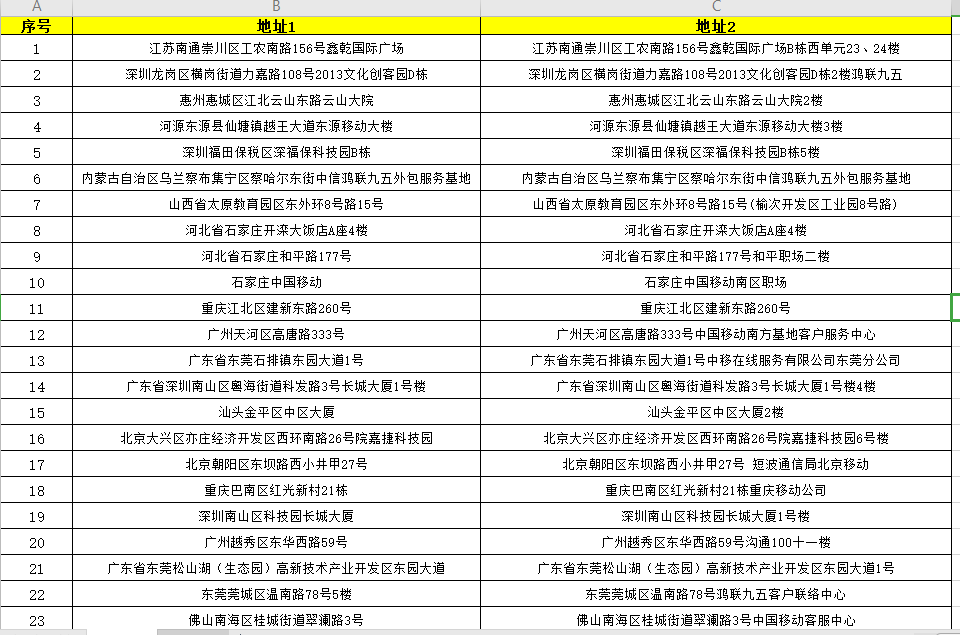2021-07-27 11:16

# 将获取到的地址信息跟Excle表中的所有地址信息做相似度计算• 点赞
• 收藏

#### 4条回答默认 最新

•Jason Ho 2021-07-27 11:47
已采纳
``````
# -*- coding: utf-8 -*-
import jieba
import numpy as np
import re

def get_word_vector(s1,s2):
"""
:param s1: 句子1
:param s2: 句子2
:return: 返回句子的余弦相似度
"""
# 分词
cut1 = jieba.cut(s1)
cut2 = jieba.cut(s2)
list_word1 = (','.join(cut1)).split(',')
list_word2 = (','.join(cut2)).split(',')

# 列出所有的词,取并集
key_word = list(set(list_word1 + list_word2))
# 给定形状和类型的用0填充的矩阵存储向量
word_vector1 = np.zeros(len(key_word))
word_vector2 = np.zeros(len(key_word))

# 计算词频
# 依次确定向量的每个位置的值
for i in range(len(key_word)):
# 遍历key_word中每个词在句子中的出现次数
for j in range(len(list_word1)):
if key_word[i] == list_word1[j]:
word_vector1[i] += 1
for k in range(len(list_word2)):
if key_word[i] == list_word2[k]:
word_vector2[i] += 1

# 输出向量
print(word_vector1)
print(word_vector2)
return word_vector1, word_vector2

def cos_dist(vec1,vec2):
"""
:param vec1: 向量1
:param vec2: 向量2
:return: 返回两个向量的余弦相似度
"""
dist1=float(np.dot(vec1,vec2)/(np.linalg.norm(vec1)*np.linalg.norm(vec2)))
return dist1

def filter_html(html):
"""
:param html: html
:return: 返回去掉html的纯净文本
"""
dr = re.compile(r'<[^>]+>',re.S)
dd = dr.sub('',html).strip()
return dd

if __name__ == '__main__':
s1="很高兴见到你"
s2="我也很高兴见到你"
vec1,vec2=get_word_vector(s1,s2)
dist1=cos_dist(vec1,vec2)
print(dist1)

``````
点赞 打赏 评论
•喜欢摸鱼的程序员 2021-07-27 11:29

excel读取然后enumerate遍历取值嘛，使用一些计算文本相似度算法或者第三方接口
把序号和相似度存个list里，遍历结束，用index取坐标输出相似度最高的值。

点赞 打赏 评论
•得一以生 2021-07-27 11:52

public static float compare(String a, String b) {
if (a == null && b == null) {
return 1f;
}
if (a == null || b == null) {
return 0F;
}
int aLen = a.length();
int bLen = b.length();
if (aLen == 0) {
return aLen;
}
if (bLen == 0) {
return bLen;
}
int[][] values = new int[aLen + 1][bLen + 1];
for (int i = 0; i <= aLen; ++i) {
for (int j = 0; j <= bLen; ++j) {
if (i == 0) {
values[i][j] = j;
} else if (j == 0) {
values[i][j] = i;
} else if (a.charAt(i - 1) == b.charAt(j - 1)) {
values[i][j] = values[i - 1][j - 1];
} else {
values[i][j] = 1 + Math.min(values[i - 1][j - 1], Math.min(values[i][j - 1], values[i - 1][j]));
}
}
}
int distance = values[aLen][bLen];
return 1 - ((float) distance / Math.max(a.length(), b.length()));
}

点赞 打赏 评论
•11Jeffrey 2021-07-27 13:51

直接调高德地图接口解析，高德地图会给你输入的地址转化成省、市、区，甚至一个地址能获取对应的经纬度坐标，调高德地图接口解析两个地址匹配省、市、区，或者经纬度就好了

点赞 打赏 评论